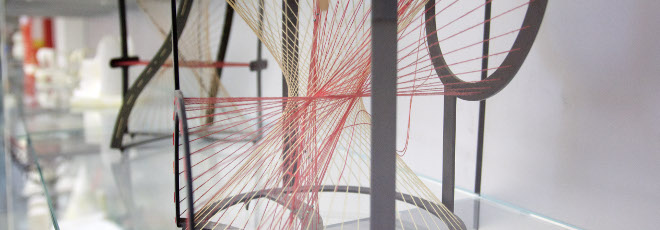## “A Well-Posed Surface Integral Equation for the Maxwell Dielectric Problem”

Mercoledì 26 Gennaio 2022, ore 17:30 - Zoom - Darko Volkov (Worcester Polytechnic Institute, USA)

Abstract

The free space Maxwell dielectric problem can be reduced to a system of surface integral equations (SIE). A numerical formulation for the Maxwell dielectric problem using an SIE system presents two key advantages: first, the radiation condition at infinity is exactly satisfied, and second, there is no need to artificially define a truncated domain. Consequently, these SIE systems have generated much interest in physics, electrical engineering, and mathematics, and many SIE formulations have been proposed over time. In this talk we introduce a new SIE formulation which is in the desirable operator form identity plus compact, is well-posed, and remains well-conditioned as the frequency tends to zero. The unknowns in the formulation are three dimensional vector fields on the boundary of the dielectric body. The resulting SIE discussed in this talk is derived from a formulation developed in earlier work. Our initial formulation utilized linear constraints to obtain a uniquely solvable system for all frequencies. The new SIE introduced and analyzed in this talk combines the integral equations from this initial formulation with new constraints. We show that the new system is in the operator form identity plus compact in a particular functional space, and we prove well-posedness at all frequencies and low-frequency stability of the new SIE.

Online Seminar on Mathematical Methods in the Theory of Electromagnetism